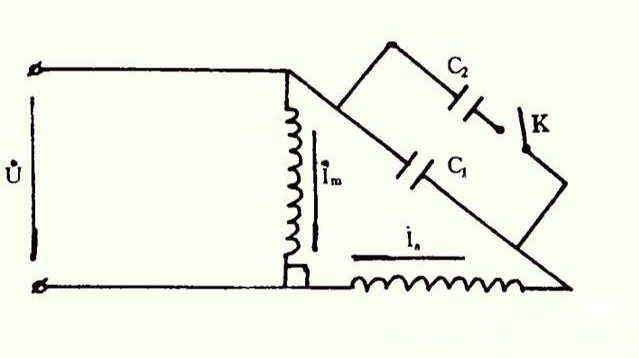News Group

# DINGFENG CAPACITOR - - Why can't start capacitor replace running capacitor

Issue Time:2020-03-23

The two capacitors of a single-phase dual-value capacitor motor, one is responsible for starting and the other is responsible for operation. This single-phase asynchronous motor has a large starting torque, high power index, small speed change, and low noise during operation.

Why use capacitors for single-phase motors?

Because AC motors must have a rotating magnetic field to rotate, while single-phase motors have only two-phase windings. The power source used is also single-phase. When power is applied, although the magnetic field is rotating, there is no starting torque, so the motor cannot turn. At this time, external force is required to start the rotor and change the original AC power by a phase difference. Connect the capacitor to the starting winding of the motor to generate the starting torque to achieve the purpose of motor rotation. This capacitor is called the startup capacitance. Therefore, as long as the capacitor is normal, the phase difference between the primary winding current and the secondary winding current of the motor can be 90 °, and a rotating magnetic field is formed in the air gap, so that the rotor can obtain the starting torque.

In a single-phase dual-value capacitor motor, a starting capacitor and a running capacitor connected in parallel with each other are connected in series in a secondary winding of the motor. When the motor starts to be energized, the parallel starting and running capacitors work together to make the motor obtain a higher starting torque, and the starting time is short. When the speed of the motor reaches 75% of the synchronous speed, the centrifugal switch on the motor shaft cuts off the connection between the starting capacitor and the secondary winding, and the running capacitor continues to be connected in series with the secondary winding of the motor and participates with the main winding of the motor jobs.The figure above is the schematic diagram of a single-phase dual-value capacitor motor, where C1 is the running capacitor, C2 is the starting capacitor, and K is the centrifugal switch. The selection of these two capacitors, first determine the capacitance value of the running capacitor, and then determine the capacitance value of the starting capacitor. The capacitance value formula of its dual-value capacitor is: C1 = 1200 × I / U × cosφ. The formula of double-value capacitor starting capacitance: C2 = (2 ～ 3) × C1.

From the above formula and capacitors in parallel, if the starting capacitor is used instead of the running capacitor, this is equivalent to two starting capacitors. 2C2 is definitely larger than C1 + C2, which is equivalent to the increase in the capacitance of the starting capacitor when the motor starts. Although the starting torque of the motor is increased at this time, the motor will increase the starting current at a larger ratio, thereby burning the motor.

In summary, it is not advisable to use a starting capacitor instead of a running capacitor in a single-phase two-value capacitor motor, which will burn the motor. In short, when the motor starts, the starting capacitor and the running capacitor work together. Only when the motor speed reaches 75% of the synchronous speed, the centrifugal switch on the motor shaft is used to cut off the starting capacitor and the auxiliary capacitor. The connection of the windings is followed by the running capacitor. Therefore, increasing the electric capacity on the basis of the original motor starting process is likely to burn out the motor.

http://www.dfcapacitor.com/

E-mail/Skype: info@dfcapacitor.com

Tel/WhatsApp: +86 15057271708

Wechat: 13857647932

Skype: Mojinxin124